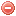# Description

The 100-hour timelag fuel moisture $$MC_{100}$$ is the moisture content of the 100-hour timelag fuels, which consist of dead roundwood in the size range of 1 to 3 inches in diameter and the forest floor from 0.75 inch to 4 inches below the surface (Deeming et al. 1977).

The calculation of the 100-hour timelag fuel moisture model requires latitude (in degrees or radians, cf. below), maximum and minimum daily air temperature [°F] and relative humidity [%] at early to midafternoon time, as well as precipitation duration during the previous 24 hours [hr] as input variables (Bradshaw et al. 1983).

NB: the calculation of the 100-hour timelag fuel moisture model requires precipitation duration (in hours) as input variable, which is not a standard meteorological variable.

# Formula

The 100-hour fuel class responds very slowly to changes in environmental conditions. Therefore, an equilibrium moisture content ($$EMC$$) representing the average drying-wetting potential of the atmosphere for the preceding 24 hours is used for its calculation (Cohen & Deeming 1985).

The 100-hour dead fuel moisture model is calculated as follows (Cohen & Deeming 1985):

First, the weighted 24-hour average $$EMC$$ is calculated:

$EMC_{24}=\dfrac{N_{nfdrs}\cdot{EMC_{min}}+(24-N_{nfdrs})\cdot{EMC_{max}}}{24}$

where $$N_{nfdrs}$$ is the daylight hours (cf. NFDRS formulation), $$EMC_{max}$$ the 24-hr maximum $$EMC$$, and $$EMC_{min}$$ the 24-hr minimum $$EMC$$. The 24-hour average $$EMC_{max}$$ is obtained by substituting $$T$$ [°F] and $$H$$ [%] by $$T_{max}$$ and $$H_{min}$$ in the standard $$EMC$$ equation (cf. Equilibrium moisture content), and the 24-hour average $$EMC_{min}$$ is obtained by substituting $$T$$ [°F] and $$H$$ [%] by $$T_{min}$$ and $$H_{max}$$ in the standard $$EMC$$ equation.

Then, the weighted 24-hour average moisture condition $$D$$ [%] is calculated as follows (Cohen & Deeming 1985):

$D=\dfrac{(24-P_{dur})\cdot{EMC_{24}}+P_{dur}\cdot(0.5\cdot{P_{dur}}+41)}{24}$

where $$P_{dur}$$ is the 24-hours precipitation duration (in hours).

Finally, the 100-hour timelag fuel moisture model $$MC_{100}$$ [%] on day i is calculated as follows (Cohen & Deeming 1985):

$MC_{100_{t}}=MC_{100_{t-1}}+(D_t - MC_{100_{t-1}})\cdot(1-0.87e^{-0.24})$

where $$MC_{100_{t-1}}$$ is the $$MC_{100}$$ value from the previous day.

The 100-hour timelag fuel moisture model is aimed to be calculated on a daily basis. The meteorological data used for its calculation have to be recorded at early to mid-afternoon time (1 to 3 pm).

NB1: The model used in the 1978 NFDRS version (cf. Burgan et al. 1977 and Deeming et al. 1977) to calculate the 100-hour timelag fuel moisture differs from the model presented here (cf. Cohen & Deeming 1985): in the 1978 version, daylength was not considered, and the 24-hour average $$EMC$$ was a function of the simple averages of the 24-hour temperature and relative humidity extremes.

NB2: If the starting value is not known or accurate, usally a starting value of 30 [%] is set. This usually allows consistent results after a four-weeks calculation period (Deeming et al. 1977).

Burgan et al. (1977)
Deeming et al. (1977)
Cohen & Deeming (1985)

### Bibliography SearchNo results!

No results were found. Are you sure you searched for a tag?

### Symbols

 Variable Description Unit $$T$$ air temperature °C $$T_{dew}$$ dew point temperature °C $$H$$ air humidity % $$P$$ rainfall mm $$U$$ windspeed m/s $$w$$ days since last rain (or rain above threshold) d $$rr$$ days with consecutive rain d $$\Delta t$$ time increment d $$\Delta{e}$$ vapor pressure deficit kPa $$e_s$$ saturation vapor pressure kPa $$e_a$$ actual vapor pressure kPa $$p_{atm}$$ atmospheric pressure kPa $$PET$$ potential evapotranspiration mm/d $$r$$ soil water reserve mm $$r_s$$ surface water reserve mm $$EMC$$ equilibrium moisture content % $$DF$$ drought factor - $$N$$ daylight hours hr $$D$$ weighted 24-hr average moisture condition hr $$\omega$$ sunset hour angle rad $$\delta$$ solar declination rad $$\varphi$$ latitude rad $$Cc$$ cloud cover Okta $$J$$ day of the year (1..365/366) - $$I$$ heat index - $$R_n$$ net radiation MJ⋅m-2⋅d-1 $$R_a$$ daily extraterrestrial radiation MJ⋅m-2⋅d-1 $$R_s$$ solar radiation MJ⋅m-2⋅d-1 $$R_{so}$$ clear-sky solar radiation MJ⋅m-2⋅d-1 $$R_{ns}$$ net shortwave radiation MJ⋅m-2⋅d-1 $$R_{nl}$$ net longwave radiation MJ⋅m-2⋅d-1 $$\lambda$$ latent heat of vaporization MJ/kg $$z$$ elevation m a.s.l. $$d_r$$ inverse relative distance Earth-Sun - $$\alpha$$ albedo or canopy reflection coefficient - $$\Delta$$ slope of the saturation vapor pressure curve kPa/°C $$Cc$$ cloud cover eights $$ROS$$ rate of spread m/h $$RSF$$ rate of spread factor - $$WF$$ wind factor - $$WRF$$ water reserve factor - $$FH$$ false relative humidity - $$FAF$$ fuel availability factor - $$PC$$ phenological coefficient -

 Suffix Description $$-$$ mean / daily value $$_{max}$$ maximum value $$_{min}$$ minimum value $$_{12}$$ value at 12:00 $$_{13}$$ value at 13:00 $$_{15}$$ value at 15:00 $$_{m}$$ montly value $$_{y}$$ yearly value $$_{f/a}$$ value at fuel-atmosphere interface $$_{dur}$$ duration $$_{soil}$$ value at soil level

 Constant Description $$e$$ Euler's number $$\gamma$$ psychrometric constant $$G_{SC}$$ solar constant $$\sigma$$ Stefan-Bolzmann constant